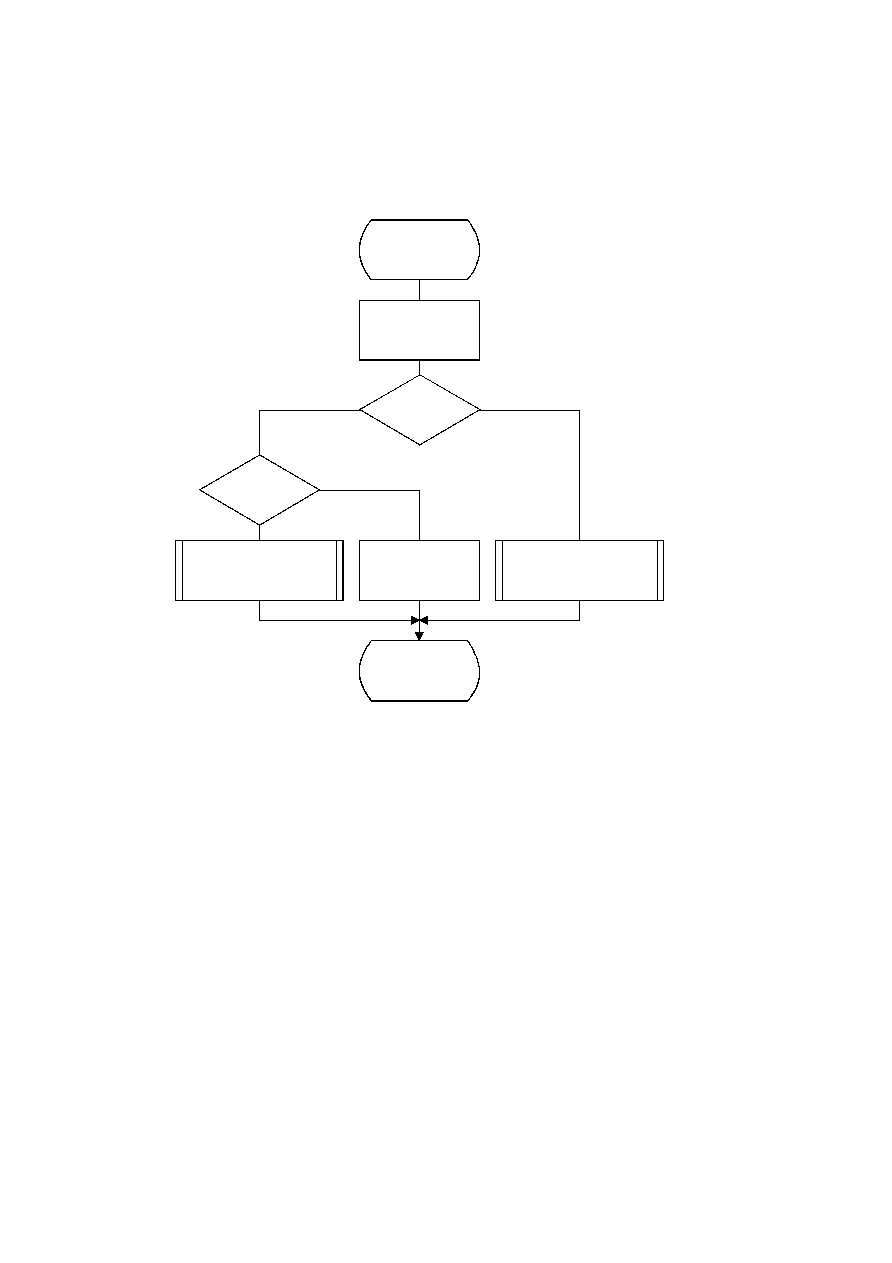ISO/IEC 10918-1 : 1993(E)
When a renormalization is needed, the MPS/LPS conditional exchange may also be needed. For the LPS path, the
conditional exchange procedure is shown in Figure D.17. Note that the probability estimation in the decoder is identical
to the probability estimation in the encoder (Figures D.5 and D.6).
TISO1160-93/d054
Decode(S)
A = A ­ Qe(S)
A < X'8000'
D = MPS(S)
Return D
Yes
No
Yes
No
D
i
=
i
Cond_MPS_exchange(S)
Renorm_d
D = Cond_LPS_exchange(S)
Renorm_d
Cx < A
?
Figure D.16 ­ Decode(S) procedure
Figure D.16 [D54], = 13.5 cm = 528.%
For the MPS path of the decoder the conditional exchange procedure is given in Figure D.18.
CCITT Rec. T.81 (1992 E)
71